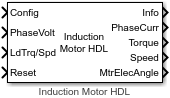# Induction Motor HDL

Three-phase induction motor

Since R2022b

•Libraries:
Motor Control Blockset HDL Support / Electrical Systems / Motors

## Description

The Induction Motor HDL block implements a three-phase induction motor. The block uses three-phase input voltages to regulate the individual phase currents, thereby controlling the motor torque or speed.

The block generates code for fixed-step double- and single-precision targets using the Sample Time (s) parameter. It supports code generation for FPGA deployment and generates HDL-compatible code.

You can specify the induction motor parameters and operating mode using the Config input port. You can also use the Induction Motor Configuration block to generate the required configuration signal to specify at the Config input port.

### Three-Phase Sinusoidal Model Electrical System

The block implements equations expressed in the stationary rotor reference (dq) frame. The d-axis aligns with the a-axis. All quantities in the rotor reference frame are referred to as the stator.The block uses these equations to calculate the electrical speed (ωem) and slip speed (ωslip).

To calculate the dq rotor electrical speed with respect to the rotor A-axis (dA), the block uses the difference between the stator a-axis (da) speed and the slip speed:

To simplify the equations for the flux, voltage, and current transformations, the block uses a stationary reference frame:

`$\begin{array}{l}{\omega }_{da}=0\\ {\omega }_{dA}=-{\omega }_{em}\end{array}$`

CalculationEquation
Flux

Current

Inductance

Electromagnetic torque

Power invariant dq transformation to ensure that the dq and the three phase powers are equal

The equations use these variables.

 ωm Angular velocity of the rotor (rad/s) ωem Electrical rotor speed (rad/s) ωslip Electrical rotor slip speed (rad/s) ωsyn Synchronous rotor speed (rad/s) ωda dq stator electrical speed with respect to the rotor a-axis (rad/s) ωdA dq stator electrical speed with respect to the rotor A-axis (rad/s) Θda dq stator electrical angle with respect to the rotor a-axis (rad) ΘdA dq stator electrical angle with respect to the rotor A-axis (rad) Lq, Ld q- and d-axis inductances (H) Ls Stator inductance (H) Lr Rotor inductance (H) Lm Magnetizing inductance (H) Lls Stator leakage inductance (H) Llr Rotor leakage inductance (H) vsq, vsd Stator q- and d-axis voltages (V) isq, isd Stator q- and d-axis currents (A) λsq, λsd Stator q- and d-axis flux (Wb) irq, ird Rotor q- and d-axis currents (A) λrq, λrd Rotor q- and d-axis flux (Wb) va, vb, vc Stator voltage phases a, b, c (V) ia, ib, ic Stator currents phases a, b, c (A) Rs Resistance of the stator windings (Ohm) Rr Resistance of the rotor windings (Ohm) P Number of pole pairs Te Electromagnetic torque (Nm)

### Mechanical System

The motor angular velocity is given by:

`$\begin{array}{c}\frac{d}{dt}{\omega }_{m}=\frac{1}{J}\left({T}_{e}-{T}_{f}-F{\omega }_{m}-{T}_{m}\right)\\ \frac{d{\theta }_{m}}{dt}={\omega }_{m}\end{array}$`

The equations use these variables.

 J Combined inertia of motor and load (kgm^2) F Combined viscous friction of motor and load (N·m/(rad/s)) θm Motor mechanical angular position (rad) Tm Motor shaft torque (Nm) Te Electromagnetic torque (Nm) Tf Motor shaft static friction torque (Nm) ωm Angular mechanical velocity of the motor (rad/s)

### Power Accounting

For motor power accounting, the block implements these equations.

Bus Signal DescriptionVariableEquations

`PwrInfo`

`PwrMtr`

Mechanical power

Pmot

`PwrBus`

Electrical power

Pbus

`PwrElecLoss`

Resistive power loss

Pelec

`PwrMechLoss`

Mechanical power loss

Pmech

When Port Configuration is set to `Torque`:

When Port Configuration is set to `Speed`:

`PwrMtrStored`

Stored motor power

Pstr

The equations use these variables.

 Rs Stator resistance (Ohm) Rr Rotor resistance (Ohm) ia, ib, ic Stator phase a, b, and c current (A) isq, isd Stator q- and d-axis currents (A) van, vbn, vcn Stator phase a, b, and c voltage (V) ωm Angular mechanical velocity of the rotor (rad/s) F Combined viscous damping of motor and load (N·m/(rad/s)) Te Electromagnetic torque (Nm) Tf Combined friction torque of motor and load (Nm)

## Ports

### Input

expand all

Configuration signal for the Induction Motor HDL block containing the block configuration parameters.

Data Types: `single`

Stator terminal voltages, Va, Vb, and Vc, in V.

Data Types: `single`

The port supports one of these inputs:

• Tm — Load torque on the motor shaft, in N·m.

• ωm — Angular velocity of the motor, in rad/s.

Data Types: `single`

Reset bus signal that the block uses to reset the internal integrators.

Data Types: `Boolean`

### Output

expand all

The bus signal contains these block calculations.

Signal DescriptionVariableUnits

`IaStator`

Stator phase current A

ia

A

`IbStator`

Stator phase current B

ib

A

`IcStator`

Stator phase current C

ic

A

`IdSync`

Direct axis current

id

A

`IqSync`

iq

A

`VdSync`

Direct axis voltage

vd

V

`VqSync`

vq

V

`MtrSpd`

Angular mechanical velocity of the rotor

ωm

`MtrMechPos`

Rotor mechanical angular position

θm

`MtrPos`

Rotor electrical angular position

θe

`MtrTrq`

Electromagnetic torque

Te

N·m

`PwrInfo`

`PwrTrnsfrd`

`PwrMtr`

Mechanical power

Pmot

W
`PwrBus`

Electrical power

Pbus

W

`PwrNotTrnsfrd`

`PwrElecLoss`

Resistive power loss

Pelec

W
`PwrMechLoss`

Mechanical power loss

Pmech

W

`PwrStored`

`PwrMtrStored`

Stored motor power

Pstr

W

Current for phase a, b, and c, ia, ib, and ic, respectively, in A.

Motor torque, Tmtr, in N·m.

Angular speed of the motor, ωmtr, in rad/s.

Electrical position of the motor, θdq, in rad.

Data Types: `single`

## Parameters

expand all

The fixed time interval (in seconds) between consecutive instances of block execution. Alternatively, you can use `-1`, to inherit the sample time from the input signals.

## Version History

Introduced in R2022b